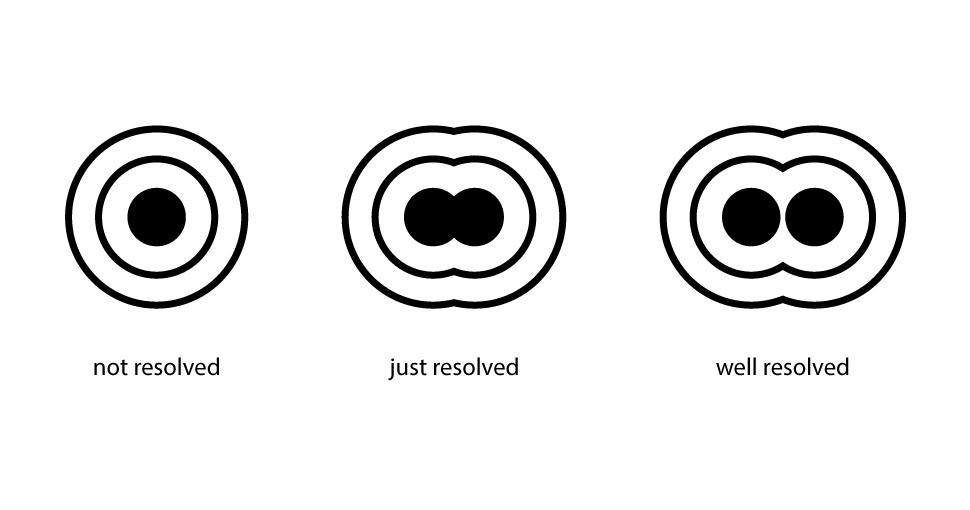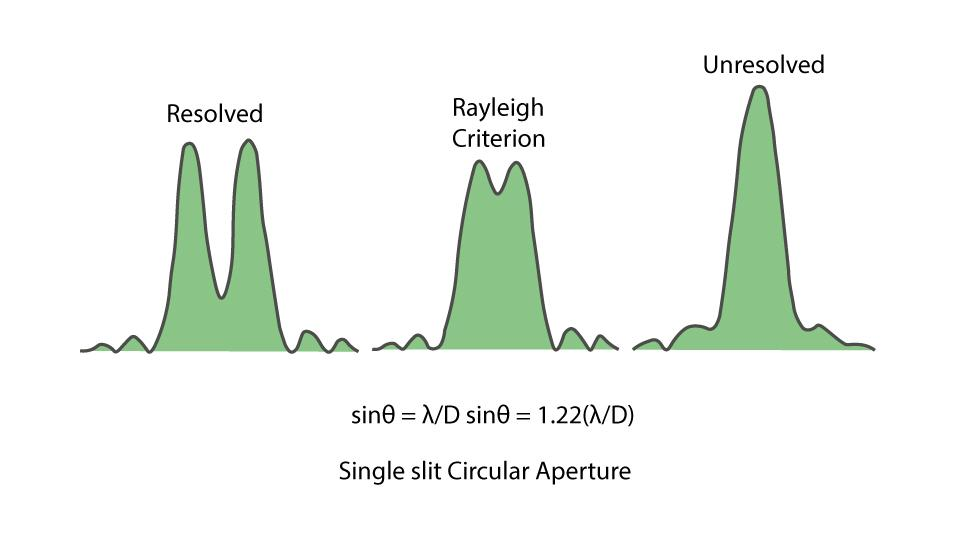Open in App
Not now

# Resolving Power of a Microscope and Telescope

• Last Updated : 03 Nov, 2022

Wave optics, also known as Physical optics, deals with the study of various phenomena such as diffraction, polarization, interference, resolution, and other occurrences. Wave optics is the segment of optics that focuses on the study and behavior of light and its wave characteristics. Wave optics particularly describes the connection between waves and rays of light. According to the wave theory of light, light is a form of energy, it travels through a medium in the form of transverse wave motion. The speed of light traveling through a medium depends upon the nature of the medium.

Here, the approximation is carried out by using ray optics for the estimation of the field on a surface. Integrating ray-estimated field over a mirror, lens, or aperture for the calculation of the scattered or transmitted field also gets involved later on. Wave optics stands as a witness to the scientific study of understanding the nature of light. One is the particle nature of light and the other is the wave nature of light.

## What is Resolving Power?

The resolving power of an optical instrument is defined as the capacity of the instrument to distinguish between two objects that are close together and produce distinct images of the two objects.

In other words, resolving power changes in inverse proportion to the distance between the two objects to be resolved when viewed from an optical instrument. The images of two close-lying objects appear distinct and separate when viewed from the device. When a telescope is used to view two stars located close to each other; the telescope’s resolving power will depend on its capacity to resolve the images of the two stars. The resolving power of a lens used in a telescope can be determined by its ability to differentiate two lines or points in an object.

## Resolving Power of Optical Instrument

Human eyes are not much powerful. They need some help in some cases. This is where optical instruments come into play. These instruments have a major role in various scientific fields. From the depths of space to the layers of hair, to see all the details we require these instruments, as we cannot see them using the naked eye. One of the important aspects of optical instruments is their resolving power.

Resolving Power is a quantity that characterizes the ability of optical instruments to produce distinct images of two points of an object that are placed nearby. Devices like microscopes and telescopes have various lenses and their combination is used to generate images of different objects. Limit of resolution is defined as the smallest linear or angular distance between the two points at which their images begin to merge. The inverse to the limit of resolution is called the resolving power.

In an ideal optical system (one without aberrations), the image of a point is not a point but a central disk of light surrounded by rings, it occurs due to the diffraction of light at the edges of optical components, which are alternately dark and bright in monochromatic light and rainbow-colored in white light.

## What is Resolution?

Resolution is the quality of the image which is decided by the diffraction effect. The resolving power of an instrument depends on its resolution. The resolution of an optical instrument is defined as the smallest distance between two points that can be distinguished by the observer’s eye or the lens installed in the optical device. Scientifically, the precision with which an optical device can record or measure a specimen’s variables under observation is also known as resolution.

It is measured by the smallest distance which could be seen clearly without blur due to diffraction. The diffraction or aberration reduces the resolution of an instrument and often creates a blurred image. Diffraction is associated with the wave nature of light. Light interferes with itself when it passes through a lens and creates a ring-shaped diffraction pattern called the Airy pattern. Such a pattern is obtained only when the wavefront of the light source is spherical or plane at the aperture’s exit point.## What is Magnification?

Magnification is the process of enlarging an object only by appearance and not by actual physical size. This enlargement can be calibrated by calculated numbers known as magnification. The magnified image does not actually become large but it appears to be large. Various lenses are used for this process of magnification. This concept of magnification is used in microscopes and telescopes. The magnification in the microscope helps in the study of the structure and composition of different microbes. whereas, the magnification in the telescope is observing the stars and planets.

## Resolution Vs Magnification

Magnification is the ability to make small objects larger to see their details properly, like making microscopic organisms visible. It is the process of enlarging an object only by appearance and not by the actual physical size. Resolution is the ability to distinguish between two objects from each other. The term magnification is often confused with resolution. Resolution determines the ability of the instrumentation system to show details in the object that is being imaged. On the other hand, magnification means without high resolution may make small microbes visible. Magnification magnifies the object’s size while resolution distinguishes between two objects. Magnification doesn’t allow the observer to distinguish between two objects. In order to distinguish between two different objects under a microscope, it should be first magnified to a specified point at which the resolution becomes much more relevant. The magnification and resolution of an optical instrument are inversely related to each other. When magnification increases, the resolution decreases, and vice versa.

## Rayleigh’s Criterion

The minimum distances between images must be such that the central maximum of the first image lies on the first minimum of the second and vice versa. Such an image viewed from an optical device is calculated using Rayleigh’s criterion.When two objects placed at a distance from each other are separated by an angular separation θ, the diffraction patterns of the two objects will overlap each other. They would appear as one when the two central maxima overlap. This defines Rayleigh’s resolution criterion. It can be shown that, for a circular aperture of a given diameter, the first minimum in the diffraction pattern occurs at,

θ = 1.22(λ/D)

Where,
θ: the angular separation measured in radians
λ: the wavelength of light
D: the diameter of the aperture

## Resolving Power of Telescope

Objects such as binary stars, individual stars, distant galaxies, and planets subtend small angles on a telescope. A larger aperture is needed to resolve these small angles and improve the resolving power of the telescope. Rayleigh’s criterion can be used to determine the resolving power of the telescope. The resolving power is inversely proportional to the angular separation between two distant objects. According to Rayleigh’s resolving power of optical instruments formula,

Δθ = 1.22(λ/d)

The resolving power is the reciprocal of angular separation:

Resolving Power = 1/Δθ = 1 / (1.22λ/d) = d/1.22λ

Thus, the higher the value of d or the diameter of the aperture, the better will be the resolution of the telescope. Astronomical optical telescopes have mirror diameters as large as 10 m to obtain the best resolution.

## Resolving Power of Optical Microscope

The resolving power of a microscope determines how far apart points can be seen separately. In the case of the Microscope, The resolving power is inversely proportional to the distance between the two objects. Its resolving power can also be determined by its resolving range. The resolution limit of a microscope is the shortest distance between two nearby objects when the images formed by the microscope are properly differentiated. The smaller the distance higher is the resolving power of the microscope. Using Ernst Abbe’s criterion, the resolution power of a microscope can be given by,

Δd = λ/(2nsinθ)

Here, the resolution Δd (smallest resolvable distance between two objects) of a microscope varies with the angular aperture.

Mathematically, the resolving power of an optical microscope can be given as:

Resolving Power = 1/Δd = (2nsinθ)/λ

where,
n is the refractive index of the medium
θ is the half-angle of the lens, which depends on the focal length and diameter of the lens.
λ wavelength of light

## What is Diffraction Limit?

When we observe a point object using a circular aperture, like a lens or iris the image formed of the point object is not a point but a diffracted pattern is formed. This phenomenon is more pronounced when the size of the object is comparable to the wavelength of light, the smallest object which can be seen is termed the Diffraction Limit.

## Solved Examples on Resolving Power

Example 1: A space telescope’s primary mirror has a diameter of 3.2 m. Determine the angle between two just-resolvable point light sources. Assume the average wavelength of light as 550 nm.

Solution:

Given,

Diameter (D) = 3.2 m

Wavelength of light (λ) =550 nm = 550 x 10-9

Using Rayleigh’s criterion,

θ = 1.22 (λ/D)

θ = 1.22 (550 x 10-9 / 3.2)

= 2.097 x 10-7 m

Example 2: Assume that light of wavelength 400nm is coming from a star. The limit of resolution of a telescope whose objective has a diameter of 2.6m is.

Solution:

Given,

Diameter of Objective Telescope (D) = 2.6 m = 100 x 2.6 cm = 260 cm

The wavelength of light (λ) = 400 nm = 4 x 10-5 cm

Limit of Resolution of telescope,

dθ = 1.22λ / D = 1.22 x 4 x 10-5/ 260

Example 3: The diameter of the objective of a telescope is 1.8m. Its resolving limit for the light of wavelength 3462 A, will be

Solution:

Given,

Diameter of objective telescope (D) = 1.8 m

Wavelength of light (λ) = 3462 A = 3462 x 10-10

Resolving limit (dθ) = 1.22λ / D

= 1.22 x 3462 x 10-10 / 1.8

Example 4: The limit of resolution of the microscope, if the numerical aperture of the microscope is 0.26, and the wavelength of light used is 900 nm, is

Solution:

Given,

Numerical Aperture (A) = 0.26

Wavelength of light (λ) = 900 nm

For a microscope, the limit of resolution is given by,

X = λ/2A

= 900 / (2 x 0.26)

= 1.73 μm

Example 5: Calculate the resolving power of the microscope if its numerical aperture is 0.12 and wavelength of light is 5000 A.

Solution:

Given,

Numerical Aperture (A) = 0.12

Wavelength of light (λ) = 5000 A

Resolving Power of Microscope (RP) = 2μsinθ / λ

= 2A / λ                                  …(Since, μsinθ = Numerical Aperture)

= 2 x 0.12 / 5000 x 10-10

= 4.8 x 105 m-1

## FAQs on Resolving Power

Question 1: What is Wave Optics?

In Physics, Wave Optics is the branch of optics where the phenomenon like ray approximation of geometric optics is not valid such as diffraction, polarization or interference, etc.

Question 2: Explain the resolving power of an optical device.

The resolving power of an optical instrument is the ability of the device to distinguish between two objects that are close together and produce distinct images of these objects.

Question 3: Define the angular limit of resolution.

The angular limit of resolution or angular resolution is the angle subtended by the two distinct objects at the objective lens of the optical instrument, when these two objects are just observed as separate objects.

Question 4: What are Airy Discs?

A diffraction pattern of concentric rings which is created by circular apertures, and grows dimmer as we go away from the center. These concentric circles are known as Airy discs.

Question 5: What is the resolving power of the Human Eyes?